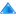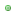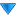# Technology type 'Mathematical Model Type'Technology TypeMathematical Model TypePartial Differential Equation (0) | Linear Equation (0) | Algebraic Equation (0) | Ordinary Differential Equation (0)

## Related assays

0 Assays visible to you, out of a total of 0
Copyright © 2008 - 2020 The University of Manchester and HITS gGmbH